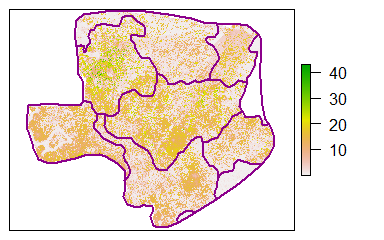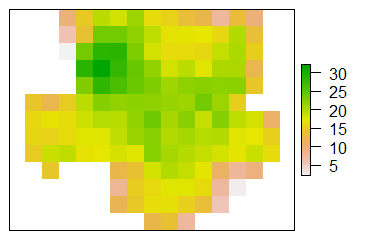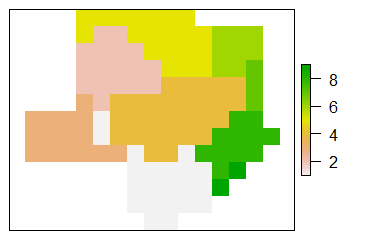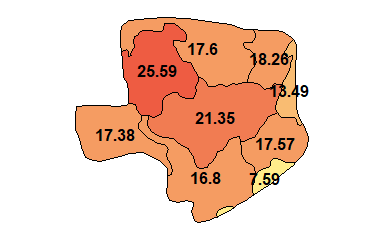# Calculating inventory attributes using Forest Tools

## Introduction

This short guide will demonstrate how to use the Forest Tools package to generate raster-based spatial statistics from a set of dominant treetops and then summarize the raster to a set of polygonal management units.

The analysis workflow is as follows:

1. Dominant treetops are detected from a canopy height model.
2. The average height of the 100 tallest trees is calculated for each 1 ha cell in a continuous grid. This metric will be referred to as top height.
3. The average top height is upscaled a second time to a set of polygonal management units (blocks).

The process for detecting dominant treetops (step 1) is explained in detail in the accompanying Canopy analysis vignette. In this document, we will simply use an existing set of treetops.

Begin by loading the Forest Tools library and sample data. The sample data is derived from a photogrammetric survey of a 125 ha area in the Quesnel Timber Supply Area in British Columbia, Canada.

# Attach the 'ForestTools' and 'raster' libraries
library(ForestTools)
library(raster)

# Load sample canopy height model, treetops and block boundaries
data("quesnelCHM", "quesnelTrees", "quesnelBlocks")

View the canopy height model (CHM) and the polygonal management units (blocks) using the plot function.

# Remove plot margins (optional)
par(mar = rep(0.5, 4))

# Plot CHM and blocks (extra optional arguments remove labels and tick marks from the plot)
plot(quesnelCHM, xlab = "", ylab = "", xaxt='n', yaxt = 'n')
plot(quesnelBlocks, add = TRUE, border =  "darkmagenta", lwd = 2)## Compute top height for 1 hectare grid

Given the large number of treetops in the quesnelTrees dataset, visualizing it in its entirety is difficult. Instead, it is more practical to view a rasterized representation of the trees’ attributes. This can be done using the sp_summarise function.

The first step is to define a function for computing the statistic that we’re interested in. In this case, we want the average height of the tallest 100 trees. It is important, however, that our function accept a number of trees lower than 100 without returning an error—in this case, it should simply return the average height of all trees in the area. We should also consider the possibility that some trees will have NA (non-available) height values.

# Create custom function for computing top height
topHgtFun <- function(x, ...) mean(tail(sort(x), 100))

In this example x would be a series of tree heights. The sort function will arrange them in ascending order (and also remove NA values), and the tail function will subset the last 100 values (i.e.: the highest values). Finally, the mean function will compute the average.

We will now apply the newly created topHgtFun to our quesnelTrees dataset using the sp_summarise function. We set the grid argument to 100 to generate a 100 m x 100 m (1 ha) grid. It is important to enter topHgtFun as a named list: “Top100” will be part of the name given to the product of the function. If other statistics are required, their corresponding functions can be added to this list.

# Use sp_summarise to generate gridded statistics
sptStatRas <- sp_summarise(trees = quesnelTrees, variables = "height", grid = 100, statFuns = list(Top100 = topHgtFun))

# View information about the result
sptStatRas
## class      : RasterBrick
## dimensions : 13, 15, 195, 2  (nrow, ncol, ncell, nlayers)
## resolution : 100, 100  (x, y)
## extent     : 492863, 494363, 5820051, 5821351  (xmin, xmax, ymin, ymax)
## crs        : +proj=utm +zone=10 +datum=WGS84 +units=m +no_defs
## source     : memory
## names      : TreeCount, heightTop100
## min values :  1.000000,     2.345474
## max values : 256.00000,     32.17429

The product of sp_summarise is a RasterBrick, i.e.: a multi-layered raster. It contains two layers: TreeCount and heightTop100, the product of our custom function when applied to the height attribute of the trees in each cell. We can subset a single layer from the RasterBrick using the [[]] operator

# Subset top height raster
topHgtRas <- sptStatRas[["heightTop100"]]

# View top height on a 1 ha grid
plot(topHgtRas, xlab = "", ylab = "", xaxt='n', yaxt = 'n')## Compute average cell height for polygonal areas

Once our raster-based statistic has been generated, there are a variety of ways summarizing the raster to a set of polygons. Note that this is different than calculating top height for the polygons directly. In the following example, the rasterize and zonal functions from the raster package are used.

# Rasterize block boundaries
blockRas <- rasterize(quesnelBlocks, topHgtRas)

# View results
plot(blockRas, xlab = "", ylab = "", xaxt='n', yaxt = 'n')# Use rasterized block boundaries to compute zonal statistics
zoneStat <- zonal(topHgtRas, blockRas, 'mean')
zoneStat
##       zone      mean
##  [1,]    1 16.797915
##  [2,]    2 25.586558
##  [3,]    3 17.376639
##  [4,]    4 21.351381
##  [5,]    5 17.595078
##  [6,]    6 18.264156
##  [7,]    7 13.490486
##  [8,]    8 17.568275
##  [9,]    9  7.589338

We can now simply attach the zonal statistics to our original polygonal areas.

# Create new 'topHeight' attribute from zonal statistics
quesnelBlocks[["topHeight"]] <- zoneStat[,"mean"]

# Plot result
library(rgeos)
colRamp <- colorRampPalette(c('lightgoldenrod1', 'tomato2'))(10)
polyCols <- colRamp[as.numeric(cut(quesnelBlocks[["topHeight"]],breaks = 10))]
plot(quesnelBlocks, col = polyCols, xlab = "", ylab = "", xaxt='n', yaxt = 'n')
text(gCentroid(quesnelBlocks, byid = TRUE), round(quesnelBlocks[["topHeight"]],2), font = 2)